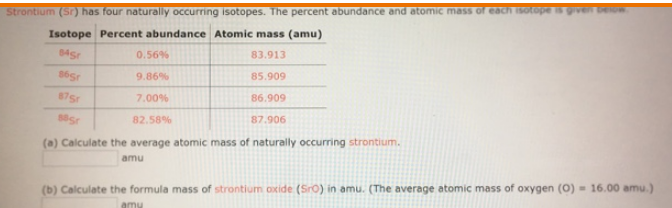# Problem: Strontium (Sr) has four naturally occurring isotopes. The percent abundance and atomic mass of each isotope is given below. (a) Calculate the average atomic mass of naturally occurring strontium. amu (b) Calculate the formula mass of strontium oxide (SrO) in amu. (The average atomic mass of oxygen (O) = 16.00 amu.) amu

###### FREE Expert Solution
92% (75 ratings)###### Problem Details

Strontium (Sr) has four naturally occurring isotopes. The percent abundance and atomic mass of each isotope is given below.

(a) Calculate the average atomic mass of naturally occurring strontium. amu

(b) Calculate the formula mass of strontium oxide (SrO) in amu. (The average atomic mass of oxygen (O) = 16.00 amu.) amu